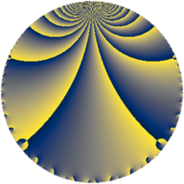Properties

 Label 1859.4.oLevel $1859$ Weight $4$ Character orbit 1859.o Rep. character $\chi_{1859}(934,\cdot)$ Character field $\Q(\zeta_{12})$ Dimension $1808$ Sturm bound $728$

Related objects

Defining parameters

 Level: $$N$$ $$=$$ $$1859 = 11 \cdot 13^{2}$$ Weight: $$k$$ $$=$$ $$4$$ Character orbit: $$[\chi]$$ $$=$$ 1859.o (of order $$12$$ and degree $$4$$) Character conductor: $$\operatorname{cond}(\chi)$$ $$=$$ $$143$$ Character field: $$\Q(\zeta_{12})$$ Sturm bound: $$728$$

Dimensions

The following table gives the dimensions of various subspaces of $$M_{4}(1859, [\chi])$$.

Total New Old
Modular forms 2240 1888 352
Cusp forms 2128 1808 320
Eisenstein series 112 80 32

Trace form

 $$1808 q + 4 q^{3} + 12 q^{4} + 8 q^{5} - 7772 q^{9} + O(q^{10})$$ $$1808 q + 4 q^{3} + 12 q^{4} + 8 q^{5} - 7772 q^{9} + 80 q^{11} - 192 q^{14} + 76 q^{15} + 13796 q^{16} - 68 q^{20} - 188 q^{22} + 240 q^{23} - 920 q^{27} + 280 q^{31} + 266 q^{33} - 2212 q^{34} + 2760 q^{36} - 328 q^{37} - 1504 q^{42} - 104 q^{44} - 896 q^{45} - 4 q^{47} - 1148 q^{48} + 12 q^{49} + 8784 q^{53} - 586 q^{55} + 1356 q^{56} - 1096 q^{58} + 1392 q^{59} - 4 q^{60} + 6360 q^{66} - 304 q^{67} + 12 q^{69} + 1932 q^{70} + 5076 q^{71} - 8832 q^{75} - 4588 q^{80} - 58280 q^{81} + 7716 q^{82} - 5608 q^{86} - 10152 q^{88} - 7268 q^{89} - 13536 q^{92} - 2740 q^{93} - 2728 q^{97} + 6996 q^{99} + O(q^{100})$$

Decomposition of $$S_{4}^{\mathrm{new}}(1859, [\chi])$$ into newform subspaces

The newforms in this space have not yet been added to the LMFDB.

Decomposition of $$S_{4}^{\mathrm{old}}(1859, [\chi])$$ into lower level spaces

$$S_{4}^{\mathrm{old}}(1859, [\chi]) \cong$$ $$S_{4}^{\mathrm{new}}(143, [\chi])$$$$^{\oplus 2}$$